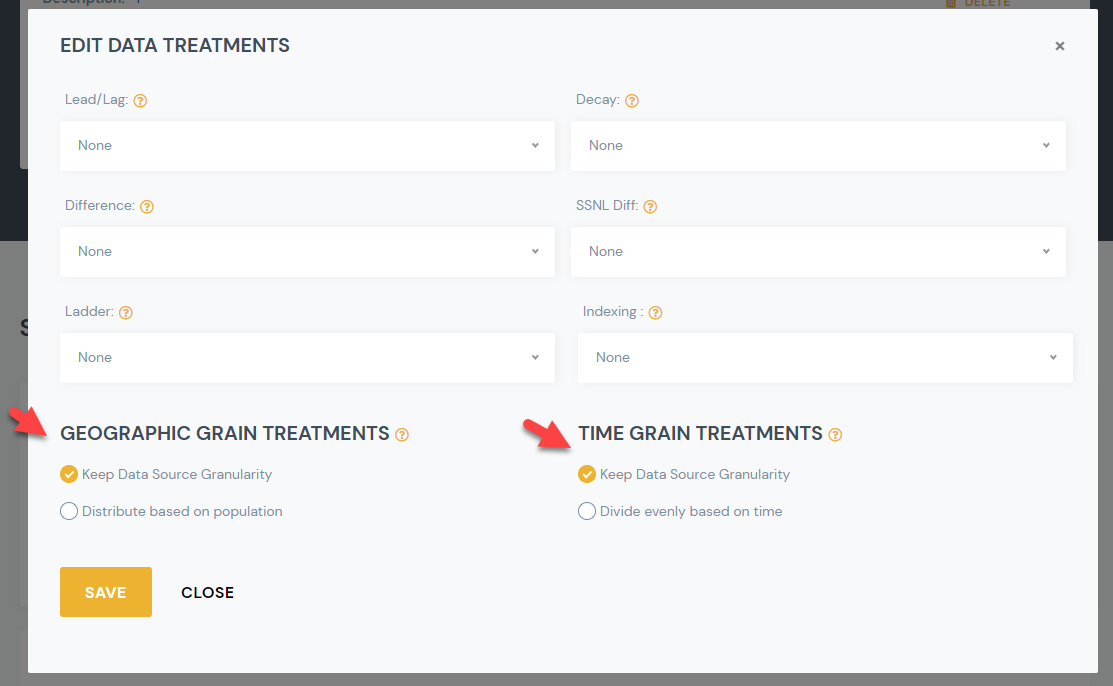Geographic and Time Grains
1. Help Center
2. Geographic and Time Grains

# Data Grains Explained

• There two types of grains that determine at what granularity data is outputted in the system:
• Time Grain: Day, Week, Month, Quarter Year
• Geographic Grain: Zip, City, County, State, Country
• Source grain vs transformed output grain.

• Source Grain is the granularity that the source data was created in.

• Output grain is the grain you want your Signal output in.

• The output grain is often not the same as the source grain.

• You have a few transformation options when moving from Source to Output grain:
• Keep Data Source Granularity (Default)
• Or Divide Evenly Based on Time (Time Grain) / Distribute Based on Population (Geo Grain)
• Your Desired Output Grain is set at the Signal Level
• This impacts the # of rows in your output and applies to all features
• You can set the desired Time & Geo grains  when creating or editing a Signal
• The Output Transformation settings are set at a Feature Level
• You can have one feature where you Keep Data Source Granularity and another where you distribute based on population.
• You can edit this value from the Manage Signal Details Page > Edit Treatments  Link
• Read below for more detail on how these "Grain transformation" options work.• For Time Grain Treatments:

• Keep Data Source Granularity (Default):

• When the time source grain is different that the Time output grain, we repeat the same value by default.

• Examples

• Source grain is “monthly”, wtih a value of 10K, but the desired output grain is daily. We would show 10K for each day.

• Source gain is “yearly”, value 8%, but output is weekly, we would show 8% for each week.

• “Divide evenly based on time” (Optional):

• This treatment allows you to spread a value evenly over a period of time. RS will do the simple algebra to spread it evenly.

• If the value is a % you cannot divide it evenly, so even if you select divide evenly we will duplicate the number.

• Example:

• Unemployment rate of 9%,  has a monthly source grain. We will show as 9% at a daily grain regardless of time grain treatment.

• If a value is a whole number, a total, then it can be divided evenly based over time.

• Example:

• New Home Sales where 30K in March.

• Output = Daily Grain: Value for each day would be 1K (30K/30Days)

• Output = Weekly Grain: Value for each week would be 7K

• For Geo Grain Treatments:
• Keep Data Source Granularity:
• When Source Granularity is LARGER than the Output granularity:
• We repeat the same value.
• Examples
• Source grain is “state”, value w 10K, but output is city. We would show 10K for each city.
• Source grain is “country”, value is 9%, but output is state. We would show 9% for each state.
• When Source Granularity is SMALLER than the Output granularity:
• If it’s a whole number, we do sum to the output granularity
• Example:  Source grain zip but output is state.  We sum to state.
• If it’s a Percentage, we take the weighted average
• Example: Source grain zip but output is state.  We take the weighted average to state [sum((percentage * population))/sum(population)].
• “Divide evenly based on population” (Optional)
• This treatment allows you to spread a value over different geographies based on population levels.
• If the value is a % you cannot spread it out, so even if you select divide evenly based on population we will duplicate the number.
• Example:
• Unemployment rate of 9%,  has a state source grain. We will show as 9% at a zip code level for all zips in that state.
• If a value is a whole number, a total, then it can be divided proportionately based on population.
• Example:
• If that input is at the state level and the output is at the zip level.  [State level value]*(Zip Population /State Population)